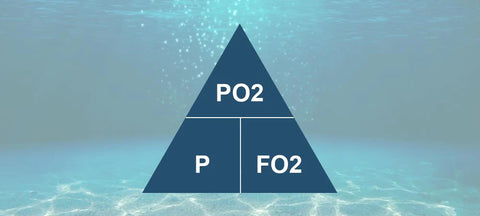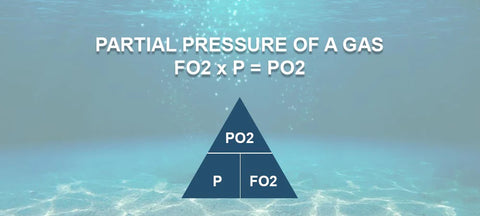# A Step by Step Guide to Nitrox Formulas## Your Guide to Pressure Triangle or Daltons Triangle

As recreational divers we are told to always keep the partial pressure (PO2) to below 1.4 ata when you dive. If you are already a nitrox diver, then some of this should be familiar to you, if you are not a  then this may not all make sense yet so get yourself booked in.

During your training your instructor may have introduced you to “The Pressure Triangle”. This is not required, but it is a useful way explain what is happening on your dive computer. This is something here at Dive Rutland, we talk about on our Nitrox courses as it useful to know how the Pressure Triangle works. We can then use that to work out some really useful information before your dive, even without having a dive computer to hand.

On Your enriched air course you will have been reminded that whilst oxygen is critical to life, it can become toxic under (partial) pressure, measured as PPO2. On regular air (21% oxygen, 79% hydrogen), toxicity typically starts below around 66m / 220ft. This is when it becomes dangerous to our Central Nervous System (CNS) and the length of exposure really comes into play.

When diving with nitrox, a diver is exposed to a higher gas partial pressure than normal, which needs to be monitored carefully. Generally speaking, recreational divers stick to a maximum PPO2 of 1.4. This number is important to remember, because it guides us in monitoring the limits of a given nitrox blend.

With this in mind, we can easily calculate what combination of Nitrox, Depth and PPO2 is safe to dive with. Dalton’s Triangle (created by John Dalton in 1802) helps us with this.

Want to find out more about Enriched Air then have a read of our

LET'S GET STARTED

These should answer most answers you have, but we understand that there are always specific questions about unique circumstances, our team can help, so reach out to them.#### USING THE PRESSURE TRIANGLE

The Pressure T, Pressure Triangle or Dalton's Triangle can be used to help you answer a number of questions you may have as a nitrox diver. The three things that the pressure triangle can give you is:

• The best mix for a given depth on a dive
• The partial pressure of the gas
• The maximum depth a gas/mix can safely be used at

Although it may look a little complicated at first, it is really pretty straight forward. You simply cover up the item you are looking for the answer to and you can find the formula you need. However, you need to understand what the letters mean:

• PO2 = Partial Pressure of Oxygen
• P = Pressure in Bar
• FO2 = Fraction of oxygen in the blend

Then you can see that there are three formulas in the above pressure triangle:

• P = PO2 / F02
• PO2 = FO2 x P
• FO2 = PO2 / P

If you are following this, then great, if not, don't worry, we are about to explain each in detail#### MAXIMUM OPERATING DEPTH FORMULA

This allows you to establish the maximum depth a gas can be safely breathed during a dive. This is worked out as follows:

• Partial Pressure of Oxygen / Fraction of Oxygen = Maximum Depth

For a dive where we do not want to exceed our 1.4 maximum pressure of oxygen with a 32% nitrox blend this would be your formula:

• 1.4 / 0.32 = 4.375

This is the absolute pressure therefore, this means we can dive to 33.75m with a 32% nitrox blend.

A good example of this is on a liveaboard when they top up your gas after the first dive on air and you analyse it at 29%.

This calculation will let you work out your Maximum Operating Depth (MOD) 1.4 ata and your Contingency Operating Depth (COD) substituting the 1.4 for 1.6 into the PO2 in the formula.#### PARTIAL PRESSURE OF GAS FORMULA

To find out the Partial Pressure of Oxygen (PO2) for a given depth and blend of gas you would work this out as follows:

• Fraction of Oxygen x Pressure in Bar = Pressure of Oxygen

For the same 25m wreck dive but choosing to use a 36% nitrox blend this would work out as follows:

• 0.36 x 3.5 = 1.26

This means that partial pressure for a 36% nitrox blend being breathed at 25m (3.5 bar) would be within our maximum allowable at 1.26 PO2.#### BEST MIX FORMULA

This is one of the most commonly used formulas. This allows you to choose the best nitrox mix for a given depth of dive to maximise your bottom time during your dive. This is worked out as follows:

• Partial Pressure of Oxygen / Pressure in Bar = Best Blend Of Nitrox

For a 25m wreck dive this would be 3.5 bar of pressure and the Partial Pressure of Oxygen we use as recreational divers is 1.4 for our maximum pressure of oxygen.

• 1.4 / 3.5 = 0.4

This means that diving a 40% nitrox blend at 25m would be our best choice of nitrox blend.

We would use this if we are planning to dive to a specific depth to see something in particular. Whilst we would ideally want the best (highest) FO2 to let us stay down longer, we compromise that to ensure a safe PO2.